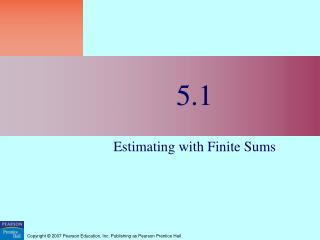# 5.1 - PowerPoint PPT PresentationDownload Presentation5.1

5.1Download Presentation## 5.1

- - - - - - - - - - - - - - - - - - - - - - - - - - - E N D - - - - - - - - - - - - - - - - - - - - - - - - - - -
##### Presentation Transcript

1. 5.1 Estimating with Finite Sums

2. Quick Review

3. Quick Review Solutions

4. What you’ll learn about • Distance Traveled • Rectangular Approximation Method (RAM) • Volume of a Sphere • Cardiac Output … and why Learning about estimating with finite sums sets the foundation for understanding integral calculus.

5. Example Finding Distance Traveled when Velocity Varies

6. Example Finding Distance Traveled when Velocity Varies

7. LRAM, MRAM, and RRAM approximations to the area under the graph of y=x2 from x=0 to x=3

8. 5.2 Definite Integrals

9. Quick Review

10. Quick Review Solutions

11. What you’ll learn about • Riemann Sums • The Definite Integral • Computing Definite Integrals on a Calculator • Integrability … and why The definite integral is the basis of integral calculus, just as the derivative is the basis of differential calculus.

12. Sigma Notation

13. The Definite Integral as a Limit of Riemann Sums

14. The Existence of Definite Integrals

15. The Definite Integral of a Continuous Function on [a,b]

16. The Definite Integral

17. Example Using the Notation

18. Area Under a Curve (as a Definite Integral)

19. Area

20. The Integral of a Constant

21. Example Using NINT

22. 5.3 Definite Integrals and Antiderivatives

23. Quick Review

24. Quick Review Solutions

25. What you’ll learn about • Properties of Definite Integrals • Average Value of a Function • Mean Value Theorem for Definite Integrals • Connecting Differential and Integral Calculus … and why Working with the properties of definite integrals helps us to understand better the definite integral. Connecting derivatives and definite integrals sets the stage for the Fundamental Theorem of Calculus.

26. Rules for Definite Integrals

27. Example Using the Rules for Definite Integrals

28. Example Using the Rules for Definite Integrals

29. Example Using the Rules for Definite Integrals

30. Average (Mean) Value

31. Example Applying the Definition

32. The Mean Value Theorem for Definite Integrals

33. The Mean Value Theorem for Definite Integrals

34. The Derivative of an Integral

35. Quick Quiz Sections 5.1 - 5.3

36. Quick Quiz Sections 5.1 - 5.3

37. Quick Quiz Sections 5.1 - 5.3

38. Quick Quiz Sections 5.1 - 5.3

39. Quick Quiz Sections 5.1 - 5.3

40. Quick Quiz Sections 5.1 - 5.3